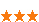您可以捐助，支持我们的公益事业。 1元 10元 50元 认证码：必填求知 文章 文库 Lib 视频 iPerson 课程 认证 咨询 工具 讲座 Modeler Code要资料订阅捐助
python数据分析与建模实现-第一次笔记427 次浏览     评价： 好 中 差
2019-7-11

 编辑推荐: 本文来自csdn ，文章主要介绍了python做数据分析时，数据的的读取和处理，以及静态结构分析等相关内容。

1.数据的读取

 （1）读取模块 Import pandas as pd Import numpy as np （2）读取表格的全部数据 df = pd.read_csv(".data/HR.csv") （3）读取你所需要的数据 sl_s=df["sactisfaction_level"]

2. 数据的处理

2.1.异常值（空值）处理

2.1.1删除

 首先，建立一个DataFrame表 1.为了确定是否含有空值： df.isnull() #如果含有空值，返回True 2.删除 df.dropna() #去掉含空值的行 如果想要删除某一个属性含空值的行就加入subset参数 df.dropna(subset=["B"]) #去掉B属性含空值的行 判断是否有重复的数据： df.duplicated(["A"]) #A属性中重复的数据返回True 删除A属性重复的行 df.drop_duplicates(["A"]) df.drop_duplicates(["A"],keep=False) #删除A属性全部重复的行 df.drop_duplicates(["A"],keep=first) #删除A属性全部重复的行，保留第一个 df.drop_duplicates(["A"],keep=last) #删除A属性全部重复的行，保留最后一个

2.1.2指代

 #含空值的数据被替换为“b*” df.fillna("b*") #E属性中的含空值的数据被替换成该属性的平均值 df.fillna(df["E"].mean()) #插值替换 如果含空值的元素为最后一个，那么空值的数据替换成和上一个数据一样 如何含空值的元素为中间，那么空值的数据被（上+下）/2代替 df["E"].interpolate() #3次样条插值 order 参数就是几次样条插值 df["E"].interpolate(method="spline",order=3)

*函数

 （4）异常值分析（含有就返回True） --isnull() sl_s.isnull() 主要表示没有空值 （5）提取异常值的该属性信息 sl_s[sl_s.isnull()] （6）提取异常值的表格全部信息 df[df["sactisfaction_level"].isnull()] （7）丢弃异常值 --dropna() sl_s=sl_s.dropna() 注：删除为空的异常值 可以利用where()把异常数据赋空，然后利用dropna()删除 （8）填充异常值 --fillna() sl_s=sl_s.fillna() （9）平均值 --mean() sl_s.mean() （10）标准差 --std() Sl_s.std() （11）最大值 --max() sl_s.max() （12）最小值 --min() sl_s.min() （13）中位数 --median() sl_s.median() （14）下四分位数 --quantile(q=0.25) sl_s.quantile(q=0.25) （15）上四分位数 --quantile(q=0.75) sl_s.quantile(q=0.75) （16）偏度 --skew() sl_s.skew() 分析：小于0 是负偏 均值偏小，大部分数是比他的均值大的 大于 0 稍微有些振偏 远大于0， 是极度振偏，均值要比他的大多数值大好多。 （17）峰度 --kurt() sl_s.kurt() 分析：<0 相比于正态分布，他的趋势相对平缓 远大于0 说明他的形变是非常大的，所以是不靠谱的 （18）获得离散化的分布(numpy模块) --histogram() np.histogram(sl_s.values,bins = np.arange(0.0,1.1,0.1)) 结果分析： [195,1214,532,974,…] [0.0,0.1,0.2,0.3,0.4…] 代表0.0-0.1之间有195个数，0.1-0.2之间有1214个数，以此类推 分布间隔为0.1

3.利用四分位数来去除异常值

 3.1.提取大于1的值 le_s[le_s>1] 3.2 去除大于1的异常值 le_s[le_s<=1] 3.3 提取正常值（利用四分位数） 3.3.1 下四分位 q_low=le_s.quantile(q =0.25) 3.3.2 上四分位 q_high=le_s.quantile(q=0.75) 3.3.3 四分位间距 q_interval=q_high-q_low 3.3.4 定义k的值 K=1.5~3之间 如果k=1.5,删除的异常值是中度异常 如果k=3.0，删除的异常值是极度异常 3.3.5 筛选 le_s=le_s[le_sq_low-k*q_interval] 3.4 数据的个数 --len() len(le_s) 3.5离散分布直方图(numpy模块) np.histogram(le_s.values,bins=np.arange(0.0,1.1,0.1)) 3.6回顾数据的平均值，标准差，中位数，最大值，最小值，偏度，峰度,确定数据的正常。

4.静态结构分析

 4.1每个值出现的次数 --values_counts() np_s.value_counts() 4.2获取该数据的构成和比例(每个值的频率) np_s.value_counts(normalize=True) 4.3 排序 np_s.value_counts(normalize=True).sort_index()

5.数据分区间

 5.1把数据分成几份 --histogram（） np.histogram(amh_s.values,bins=10) 把数据分成10份 5.2另一种方法 加了区间，计算区间的频数 (左闭右开的区间) Np.histogram(amh_s.values,bins = np.arange(amh_s.min(),amh_s.max()+10,10)) （左开右闭的区间） amh_s.value_counts(bins=np.arange (amh_s.min(),amh_s.max()+10,10))

6.英文异常值数据的处理

 6.1 首先，统计该数据的分布频数 s_s.value_counts() 6.2确定异常值的名字。 6.3把异常值赋空(NaN) --where() s_s.where(s_s!="name") 意思是把”name”的数据赋空 6.4把赋空的异常值删除 --dropna()删除异常值 s_s.where(s_s!="name").dropna() 6.5 检查删除异常值的结果 s_s.where(s_s!="name").dropna().value_counts()

7.对比分析

 7.1对表格中空值的行删除 Df = df.dropna(axis=0,how=’any’) axis =0 ,代表的是行删除 how=‘any’ 代表的是含有部分空值就执行行删除 how=‘all’ 代表的是一行全部是空值执行行删除 7.2含有条件性的对异常值的删除 df=df[df["last_evaluation"]<=1] [df["salary"]!="name"][df["department" ]!="sale"] 7.3分组(比如：把同一部门的人分为一组) --groupby() df.groupby("department") 7.4对分组后的组取均值 df.groupby("department").mean() 7.5 取部分数据（切片） --loc() df.loc[:,["last_evaluation","department"]] .groupby("department") 7.6 取部分数据求平均 df.loc[:,["last_evaluation","department"]] .groupby("department").mean() 7.7 取部分数据求极差 --apply() df.loc[:,["average_monthly_hours" ,"department"]].groupby ("department")[ "average_monthly_hours"]. apply(lambda x:x.max()-x.min())427 次浏览     评价： 好 中 差订阅捐助
 相关文章 我们该如何设计数据库 数据库设计经验谈 数据库设计过程 数据库编程总结
 相关文档 数据库性能调优技巧 数据库性能调整 数据库性能优化讲座 数据库系统性能调优系列